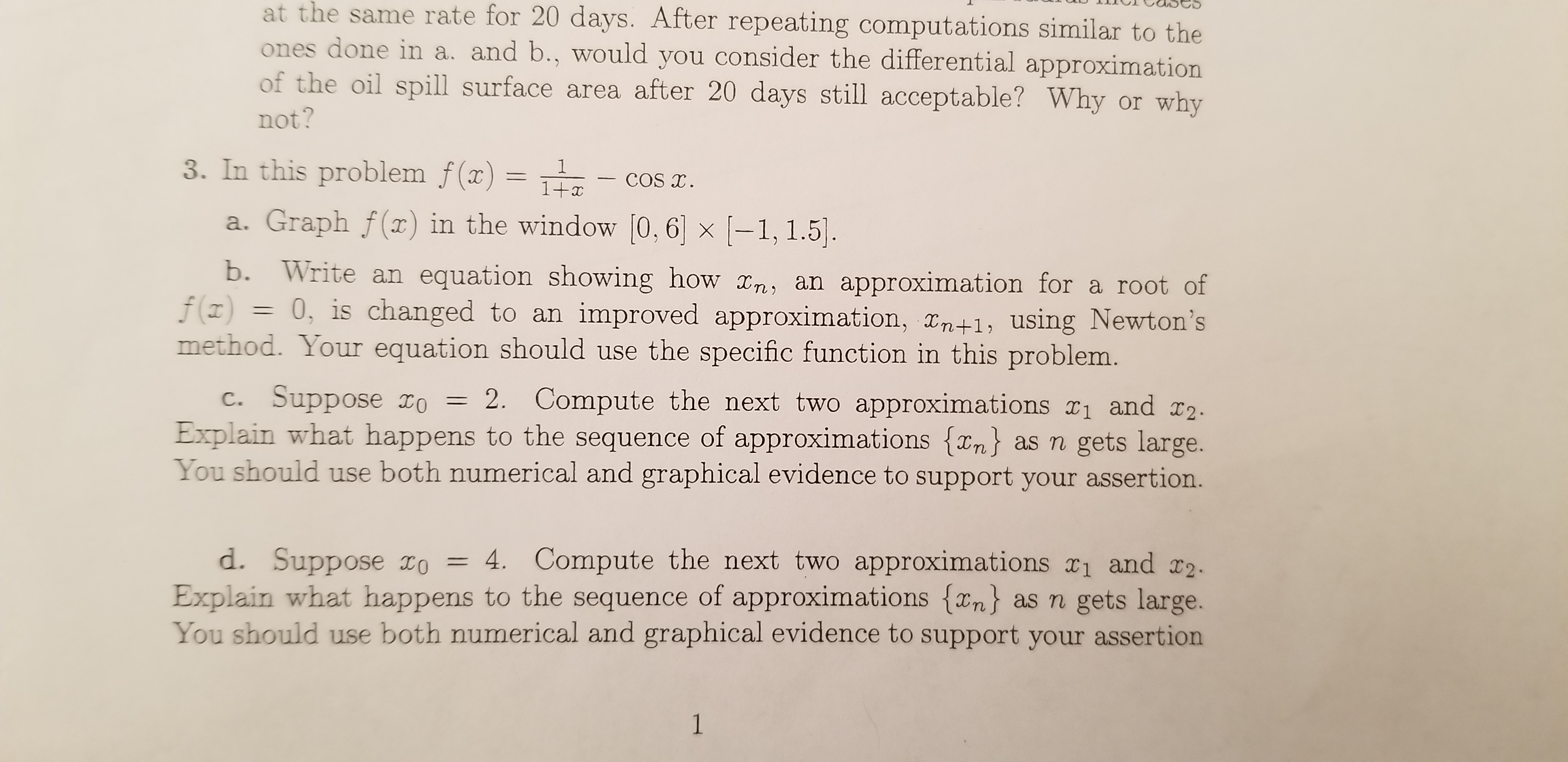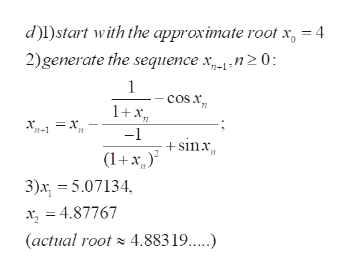# at the same rate for 20 days. After repeating computations similar to theones done in a. and b., would you consider the differential approximationof the oil spill surface area after 20 days still acceptable? Why or whynot?3. In this problem f(x) =1COS T1+xa. Graph f(x) in the window [0,6 x -1, 1.5.b. Write an equation showing how x,n, an approximation for a root off(z)0, is changed to an improved approximation, Tn+1, using Newton'smethod. Your equation should use the specific function in this problem.c. Suppose IOExplain what happens to the sequence of approximations {xn as n gets large.You should use both numerical and graphical evidence to support your assertion.2. Compute the next two approximations r1 and r2d. Suppose IO = 4. Compute the next two approximations x1 and I2.Explain what happens to the sequence of approximations {xn} as n gets large.You should use both numerical and graphical evidence to support your assertion1

Question

Question 3 part Dhelp_outlineImage Transcriptioncloseat the same rate for 20 days. After repeating computations similar to the ones done in a. and b., would you consider the differential approximation of the oil spill surface area after 20 days still acceptable? Why or why not? 3. In this problem f(x) = 1 COS T 1+x a. Graph f(x) in the window [0,6 x -1, 1.5. b. Write an equation showing how x,n, an approximation for a root of f(z) 0, is changed to an improved approximation, Tn+1, using Newton's method. Your equation should use the specific function in this problem. c. Suppose IO Explain what happens to the sequence of approximations {xn as n gets large. You should use both numerical and graphical evidence to support your assertion. 2. Compute the next two approximations r1 and r2 d. Suppose IO = 4. Compute the next two approximations x1 and I2. Explain what happens to the sequence of approximations {xn} as n gets large. You should use both numerical and graphical evidence to support your assertion 1 fullscreen
check_circleExpert Solution
Step 1

To apply Newton's method for finding approximate root for f(x)=0 with initial approximation x0=4

Step 2

Newton's algorithm yields better and better approxim...help_outlineImage Transcriptionclosed)l)start with the approximate root x = 4 2)generate the sequence xn0 n+1 1 COS 71 1+x = x. -1 - sinx (1+x 3)x 5.07134 x, = 4.87767 (actual root4.883 1....) fullscreen

### Want to see the full answer?

See Solution

#### Want to see this answer and more?

Solutions are written by subject experts who are available 24/7. Questions are typically answered within 1 hour*

See Solution
*Response times may vary by subject and question
Tagged in

### Math